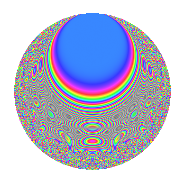# Properties

 Label 731.2.jLevel 731 Weight 2 Character orbit j Rep. character $$\chi_{731}(135,\cdot)$$ Character field $$\Q(\zeta_{6})$$ Dimension 128 Newform subspaces 1 Sturm bound 132 Trace bound 0

# Related objects

## Defining parameters

 Level: $$N$$ = $$731 = 17 \cdot 43$$ Weight: $$k$$ = $$2$$ Character orbit: $$[\chi]$$ = 731.j (of order $$6$$ and degree $$2$$) Character conductor: $$\operatorname{cond}(\chi)$$ = $$731$$ Character field: $$\Q(\zeta_{6})$$ Newform subspaces: $$1$$ Sturm bound: $$132$$ Trace bound: $$0$$

## Dimensions

The following table gives the dimensions of various subspaces of $$M_{2}(731, [\chi])$$.

Total New Old
Modular forms 136 136 0
Cusp forms 128 128 0
Eisenstein series 8 8 0

## Trace form

 $$128q - 4q^{2} + 116q^{4} - 12q^{8} + 70q^{9} + O(q^{10})$$ $$128q - 4q^{2} + 116q^{4} - 12q^{8} + 70q^{9} + 4q^{13} - 12q^{15} + 76q^{16} + 2q^{17} - 16q^{18} - 2q^{19} - 20q^{21} + 60q^{25} - 2q^{26} - 28q^{30} - 48q^{32} + 22q^{33} - 18q^{34} - 112q^{35} + 36q^{36} - 40q^{38} + 36q^{42} + 10q^{43} + 36q^{47} + 52q^{49} + 16q^{50} + 10q^{51} + 10q^{52} + 24q^{55} - 12q^{59} - 78q^{60} + 36q^{64} + 14q^{66} + 10q^{67} - q^{68} - 64q^{70} - 68q^{72} - 22q^{76} - 28q^{77} - 20q^{81} - 6q^{83} + 32q^{84} + 6q^{85} - 58q^{86} + 32q^{87} + 36q^{89} + 6q^{93} + 132q^{94} - 48q^{98} + O(q^{100})$$

## Decomposition of $$S_{2}^{\mathrm{new}}(731, [\chi])$$ into newform subspaces

Label Dim. $$A$$ Field CM Traces $q$-expansion
$$a_2$$ $$a_3$$ $$a_5$$ $$a_7$$
731.2.j.a $$128$$ $$5.837$$ None $$-4$$ $$0$$ $$0$$ $$0$$

## Hecke Characteristic Polynomials

There are no characteristic polynomials of Hecke operators in the database